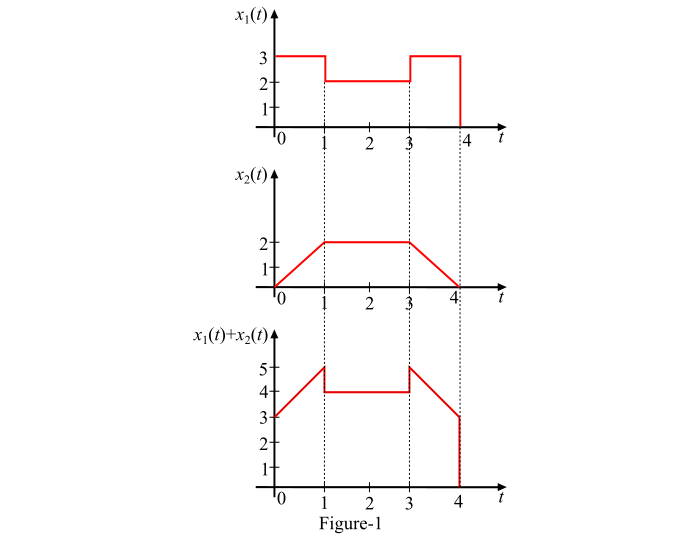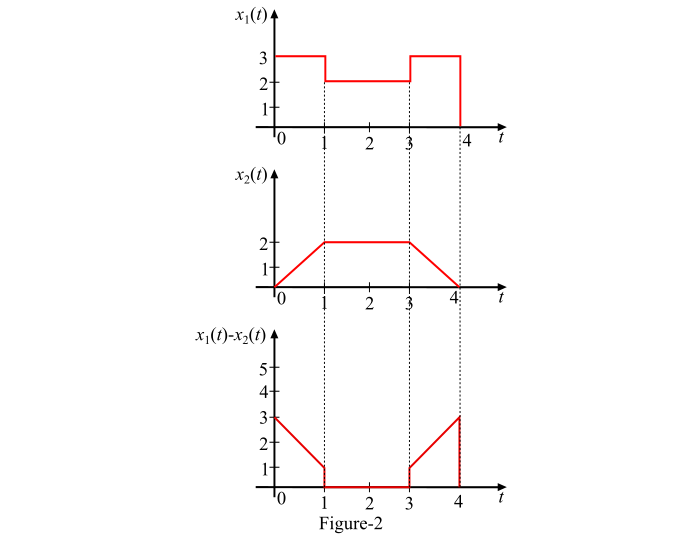# Signals and Systems: Addition and Subtraction of Signals

## Addition and Subtraction of Continuous-Time Signals

The sum of two continuous time signals 𝑥1(𝑡) and 𝑥2(𝑡) can be obtained by adding their values at every instant of time. Likewise, the difference of two continuous time signals 𝑥1(𝑡) and 𝑥2(𝑡) can be obtained by subtracting the values of one signal (say 𝑥2(𝑡)) from another signal (say 𝑥1(𝑡)) at every instant of time.

Let two continuous time signals 𝑥1(𝑡) and 𝑥2(𝑡) as shown in Figure-1. The sum of these two signals 𝑥1(𝑡) + 𝑥2(𝑡) is also shown in Figure-1.### Explanation

The sum of these two signals can be obtained by considering different time intervals as follows −

• For 𝟎 ≤ 𝒕 ≤ 𝟏− 𝑥1(𝑡) = 3 and 𝑥2(𝑡) is increasing linearly from 0 to 2. Therefore, the sum [i.e., 𝑥1(𝑡) + 𝑥2(𝑡)] will also increase linearly from (3 + 0 = 3) to (3 + 2 = 5).

• For 𝟏 ≤ 𝒕 ≤ 𝟑− 𝑥1(𝑡) = 2 and 𝑥2(𝑡) = 2, thus

𝑥1(𝑡) +𝑥2(𝑡) = 2 + 2 = 4

• For 3≤ 𝒕 ≤ 𝟒− 𝑥1(𝑡) = 3 and 𝑥2(𝑡) decreases linearly from 2 to 0. Hence, the sum of the signals will also fall linearly from (3 + 2 = 5) to (3 + 0 = 3).

## Subtraction of Signals

The subtraction [𝑥1(𝑡) − 𝑥2(𝑡)] of two continuous time signals 𝑥1(𝑡) and 𝑥2(𝑡) is shown in Figure-2.### Explanation

The difference of two continuous-time signals 𝑥1(𝑡) and 𝑥2(𝑡) can be obtained by considering different time intervals as follows −

• For 𝟎 ≤ 𝒕 ≤ 𝟏−𝑥1(𝑡) = 3 and 𝑥2(𝑡) is increasing linearly from 0 to 2. Thus, the difference [𝑥1(𝑡) −𝑥2(𝑡)] falls linearly from (3 – 0 = 3) to (3 – 2 = 1).

• For 𝟏 ≤ 𝒕 ≤ 𝟑− 𝑥1(𝑡) = 2 and 𝑥2(𝑡) = 2, thus

𝑥1(𝑡) − 𝑥2(𝑡) = 2 − 2 = 0

• For 3≤ 𝒕 ≤ 𝟒− 𝑥1(𝑡) = 3 and 𝑥2(𝑡) decreases linearly from 2 to 0. Hence, the difference of the signals will rise linearly from (3 – 2 = 1) to (3 – 0 = 3).

## Addition and Subtraction of Discrete-Time Signals

In the discrete time case, the sum of two sequences 𝑥1(𝑛) and 𝑥2(𝑛) can be obtained by adding the corresponding sample values. Similarly, the difference of the two sequences 𝑥1(𝑛) and 𝑥2(𝑛) can be obtained by subtracting each sample of one signal from the corresponding sample of the other signal.

Let two discrete-time sequences are given as follows −

𝑥1(𝑛) = {2, 1, 3, 5, 2}

𝑥2(𝑛) = {1, 4, 2, 1, −3}

Then, the addition of discrete-time signals is,

𝑥1(𝑛) + 𝑥2(𝑛) = {2 + 1, 1 + 4, 3 + 2, 5 + 1, 2 − 3} = {3, 5, 5, 6, −1}

Similarly, the subtraction of discrete-time signals is,

𝑥1(𝑛) - 𝑥2(𝑛) = {2 − 1, 1 − 4, 3 − 2, 5 − 1, 2 + 3} = {1, −3, 1, 4, 5}